Next: The Lorentz transformations Up: Kinematics of special relativity Previous: Additional remarks   Contents

# Relativity of simultaneity

Now, after criticism of the fundamental concept of time for SRT, we continue the analysis of the logical basis of this theory and consider the subsidiary notion of the "relativity of simultaneity". Recall the mental experiment from SRT: a trainpasses along a railroad at speed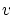. Suddenly, lightning strikes the railroad bed (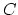) just opposite to the train center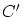(at the moment of coincidence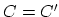). Then, in the coordinate system centered on the moving train, the flashes will simultaneously arrive at points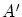and, whereas for a motionless observer the flashes will simultaneously arrive at points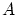and(with the middle at point); but up to this instant, pointsand(the middles of segments) will move to some distance from each other. But a similar situation is possible in classical physics as well, if we want to transmit information from pointsto the new single point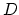(or, conversely, to these pointsfrom) at some finite speed(in this case SRT and light speed constancy will be without any relevance).

We can suggest the following mechanical model (Fig. 1.11):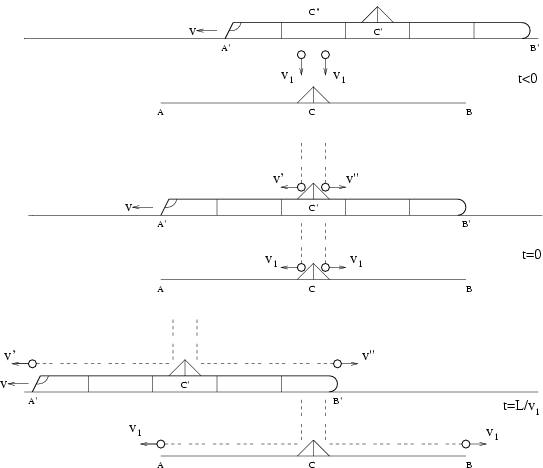Let four material points (without the force of gravity) fall at speedin pairs over point(close to the railroad bed) and over the train's centrewhich will arrive to the point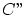near to pointat the moment of intercept of falling points. Let ideal reflectors (isosceles triangles with angle at a base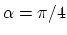) be installed at pointand at train's center. Then two particles, reflected over the railroad bed (at point), will fly to different sides at speed, and simultaneously reach pointsand(in the classics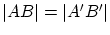). This process will take time, where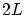is the length of the train. Two other particles, reflected over the train's center, will move after reflection (relative to the railroad) at speeds(forwards) and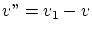(backwards). During the same time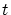the first of these particles will traverse the path (forwards)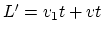, and, since the train traverses the path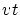, the particle will reach point. Similarly, for the second particle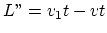; hence, it reaches point. Thus, the event - the falling of points to the reflectors - will be recorded at all four points simultaneously: both at pointsand(over the railroad bed), and at pointsand(over the train). It is the case when the points, falling over train, participated in its inertial movement. If the second pair of points falls (over the railroad bed) just over motionless pointthe triangular reflector at the train (only at it) should have the following corners at the basis: against the train movement -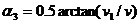, and in the direction of the train movement -. In this case particles will fly in parallel to the train and will reach its ends simultaneously (but not simultaneously with the second pair of particles!). If we want, that all four material points "have flown by" simultaneously corresponding points, corners at the reflector basis (at the train) should be still reduced by corner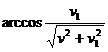(if to establish a flat waveguide, the pair of particles over the train will "not rise" too highly, and will move in parallel to the train). Apparently, mechanical analogues are possible for the most different situations.

One can say that these two events are quite different. But in the case of the light flash, we have two different events as well. Indeed, let the light flash occur at the time the centers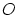andof systems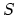andmoving relative to each other at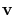coincide. At some time instant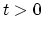, the light front will be on the sphererelative to centerin systemand on the sphere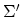with centerin system(which seems to be impossible). However, there is nothing surprising (i.e. contradicting classical physics) in this situation, because the observers in systemandwill record the same light to have different frequencies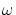and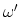by virtue of the Doppler effect. But in this case these are two identifiably different events: the observers can always compare the results of measurementsandupon meeting!

Consider now in detail the mental experiment allegedly "demonstrating" the relativity of simultaneity: at the originsandof reference systemsandthat move relative to each other, a light flash occurs at the time of their coincidence. According to SRT, during the timeon the clock of system, the light will pass the distancefrom center. For the same timeon the clock of system, the same light will pass the distance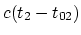from center. The time differenceis not influenced by any adjustment of initial times, whether accomplished before the experiment, or after it by any method. For example, an infinitely remote periodic source located perpendicular to the direction of motion can be used. It is possible to agree in advance about the flashes, recorded on the clock of system(for example, periodically each million years), and "to organize" systemfor one instant before the flash occurs, selected in advance (the paradox of non-locality, associated with this, will be considered in Section 1.7).

Recall that the basic positive idea of SRT consisted in the finiteness of the speed of interactions. The same idea is expressed by a short-range interaction theory, which reflects the field approach (via the Maxwell equations); namely: a light wavefront moving from a source to a receiver passes sequentially through all intermediate points of space. It is just this property that comes in a conflict with the notion of relativity of simultaneity (Fig. 1.12).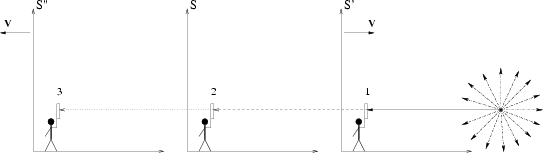To prove it, we use two statements from the SRT about observers moving each relative other: 1) one and the same light flash will reach two observers simultaneously despite the fact that the observers will spatially be separated by some distance during the light spreading; 2) kinematic formulas of the SRT (from textbooks) contain squares of velocities only. For example, let the first observer in systembe moving towards the flash source at slow speedm/s. Since the distance to the flash point is large (say a million light years), then for one million years both observers will separate from each other to a large distance - about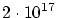m. According to SRT formulas, the times of arrival of a signal will be the same for both observers. At what point of space did the first observer "pass" the light wavefront for the second observer? But what if he had held a mirror for the whole million years, and removed it one second before receiving a signal? In the second observer's opinion, the signal was reflected by the first observer somewhere ahead. But in this case what thing was reflected by the first observer, if none of his instruments did still respond to a flash? Similarly, a third observer can go away from the second one at the same velocity, but directed from the source. If the second observer held a mirror for a million of years except one second, would the third one see the light?

On the one hand, since the SRT formulas include the square of velocity only, the second observer will consider the time of signal reception by the first and third observers to be the same. It can be agreed that when observers receive the signal under investigation, each of them will send his signal without delay. If second observer's calculations are correct, then since the problem is symmetric, he must receive the signals from the first and third observers simultaneously. On the other hand, according to Maxwell equations, the light propagates continuously, and the second observer will receive a signal from the first one simultaneously with the event, when he himself will see the signal under investigation. In second observer's opinion, at this time the light has still not reached the third observer. Thus, the second observer comes to a contradiction with himself: the first calculations by SRT formulas contradict the second calculations by the Maxwell equations. Obviously, the observers will see the flash sequentially, rather than simultaneously, since the spatial path of light is sequential: the source, the first observer, then the second and, at last, the third observer.

We additionally note that even within the SRT framework the concept of the relativity of simultaneity is highly restricted: it is applicable to two separated events only (there are no intersecting original causes, no intersecting aftereffects, and, generally, we are not interested in any additional facts). Indeed, even for these selected points the light cones have intersections, to say nothing of all other points in space and time. In fact, we have continuous chains of causally bound (and unbound) events occurring with multiple intersections through every point of space and time (not every reason, of course, results in a consequence at a speed of light). And all this real (different in scale!) time grid is interdependent for the whole space. Therefore, in the general case we can not change (by choosing the frame of reference) the order of succession of even causally unbound events (in any case, this changing would be reflected somewhere).Next: The Lorentz transformations Up: Kinematics of special relativity Previous: Additional remarks   Contents
Sergey N. Arteha